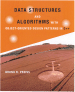Data Structures and Algorithms with Object-Oriented Design Patterns in C++Fibonacci Hashing

The final variation of hashing to be considered here is called the Fibonacci hashing method  . In fact, Fibonacci hashing is exactly the multiplication hashing method discussed in the preceding section using a very special value for a. The value we choose is closely related to the number called the golden ratio.

The golden ratio  is defined as follows: Given two positive numbers x and y, the ratio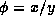is the golden ratio if the ratio of x to y is the same as that of x+y to x. The value of the golden ratio can be determined as follows: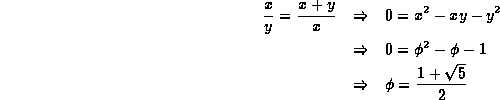There is an intimate relationship between the golden ratio and the Fibonacci numbers . In Sectionit was shown that the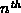Fibonacci number is given by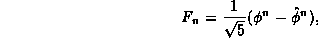where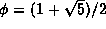and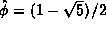!

In the context of Fibonacci hashing, we are interested not in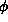, but in the reciprocal,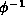, which can be calculated as follows: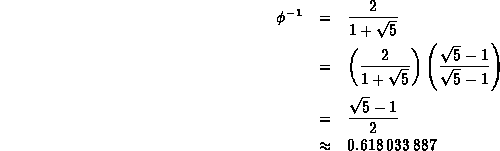The Fibonacci hashing method is essentially the multiplication hashing method in which the constant a is chosen as the integer that is relatively prime to W which is closest to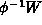. The following table gives suitable values of a for various word sizes.

 W a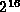40503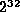2654435769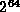11400714819323198485

Why is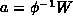special? It has to do with what happens to consecutive keys when they are hashed using the multiplicative method. As shown in Figure, consecutive keys are spread out quite nicely. In fact, when we useto hash consecutive keys, the hash value for each subsequent key falls in between the two widest spaced hash values already computed. Furthermore, it is a property of the golden ratio,, that each subsequent hash value divides the interval into which it falls according to the golden ratio!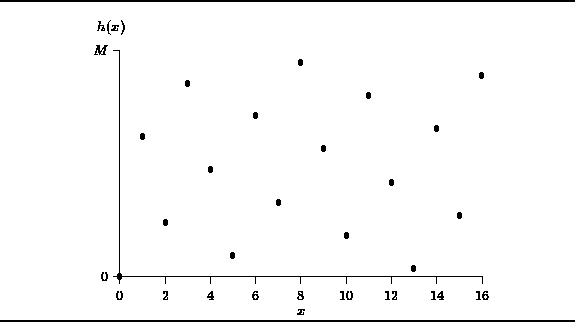Figure: Fibonacci HashingCopyright © 1997 by Bruno R. Preiss, P.Eng. All rights reserved.